Product category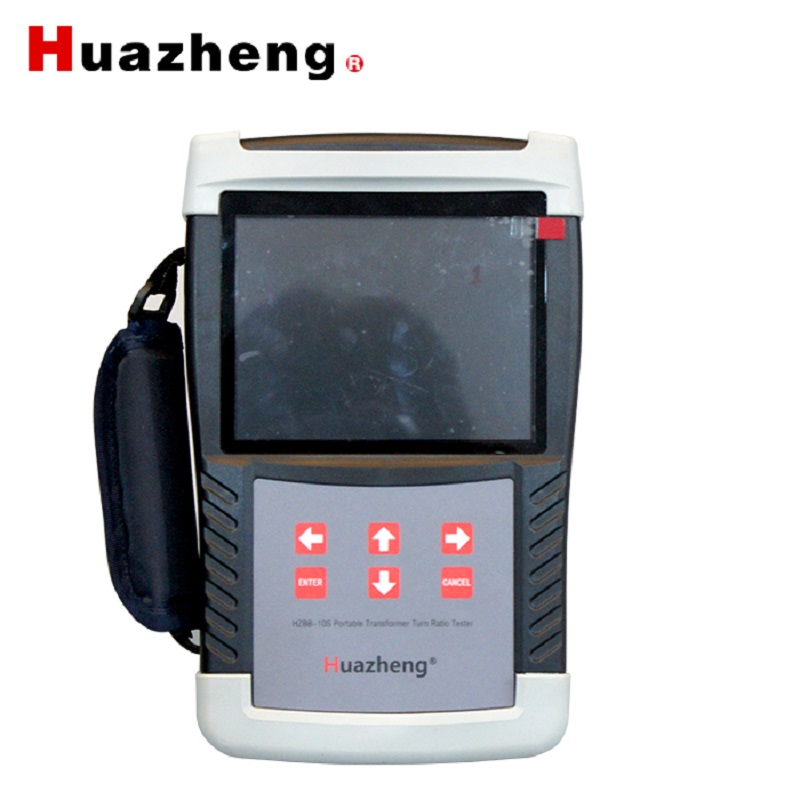HZBB-10S turns ratio tester transformer ratio testing equipment automatic transformer turns ratio meter ttr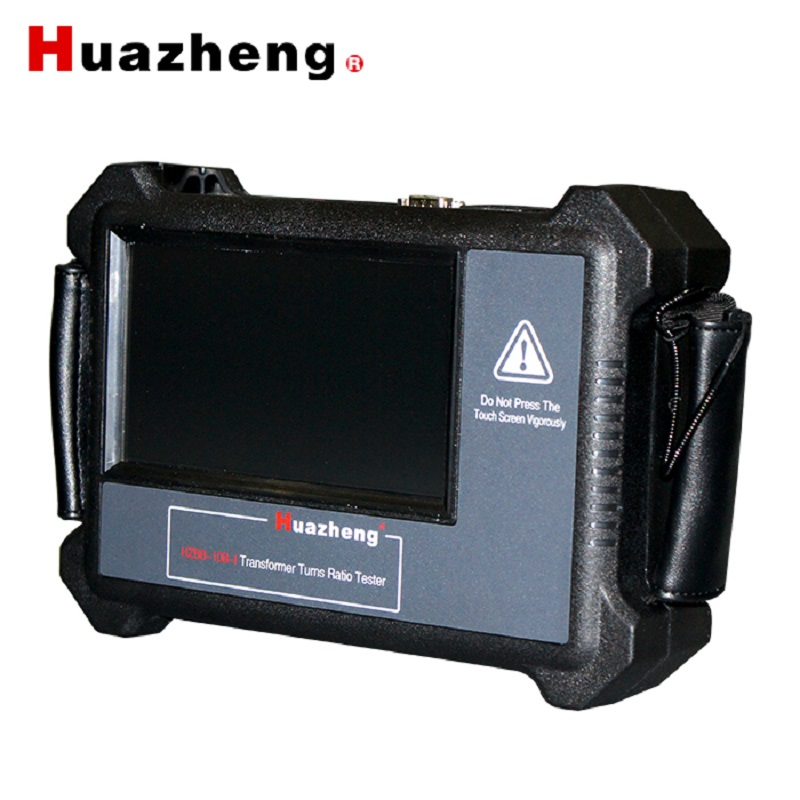HZBB-10B-I Turns Ratio Tester Turns Ratio Tester Portable TTR Meter Transformer Turns Ratio Analysis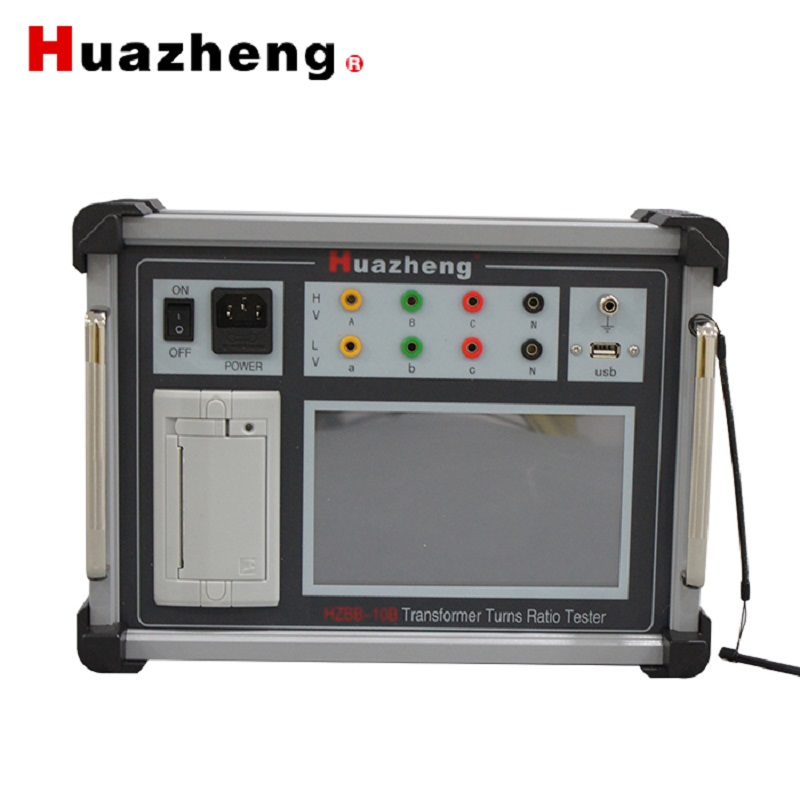HZBB-10B ttr testing equipment turns ratio tester transformer turns ratio meter ttr digital transformer turns ratio meter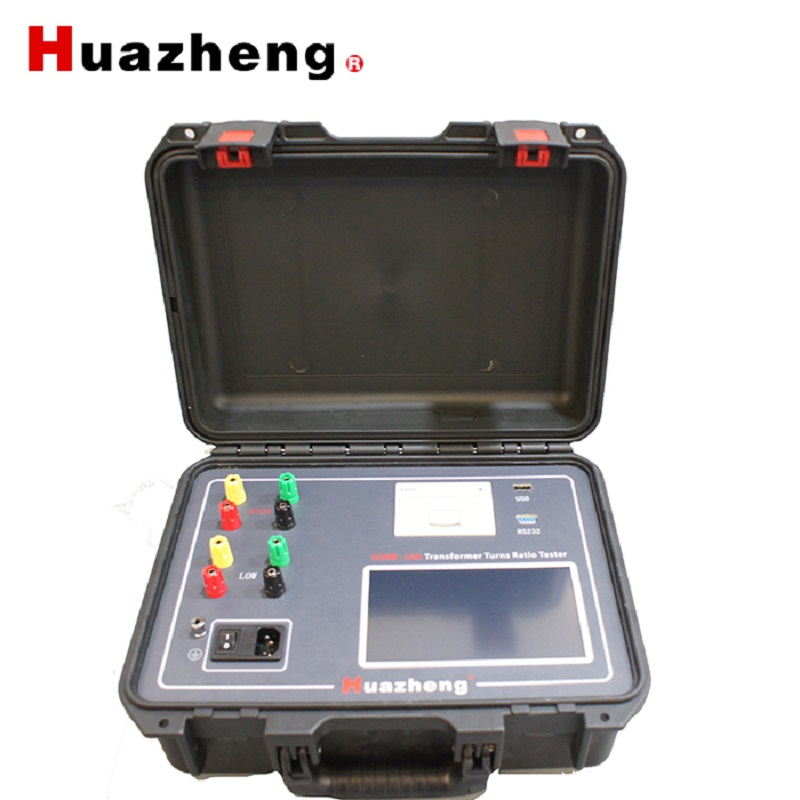Huazheng Electric HZBB-10A Turns Ratio Group Tester Turns Ratio Tester Transformer TTR Tester Equipment Electric Test Turn Ratio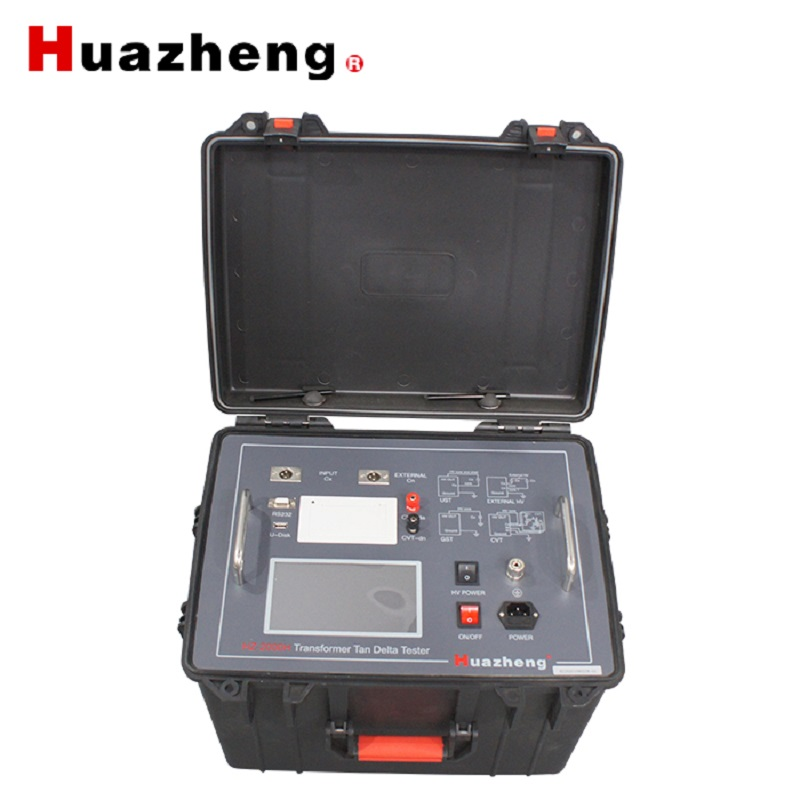HZ-2000H capacitance and dissipation factor measuring instrument transformer tan delta tester dielectric loss factor tester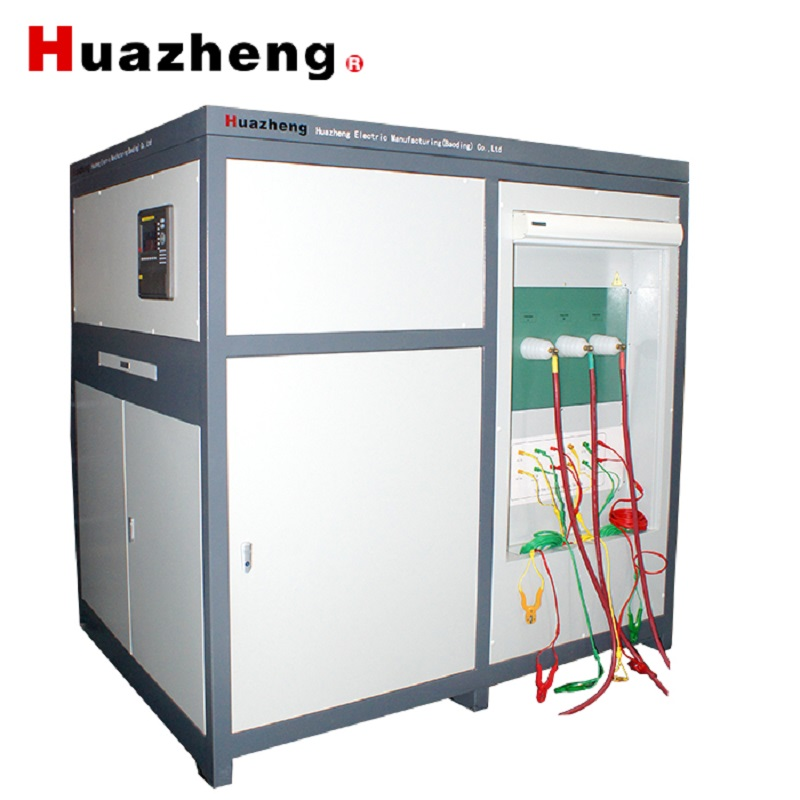Huazheng Electric HZ-IV Transfotmer Test Bench Transformer Characteristic Comprehensive Test Bench Transformer Test System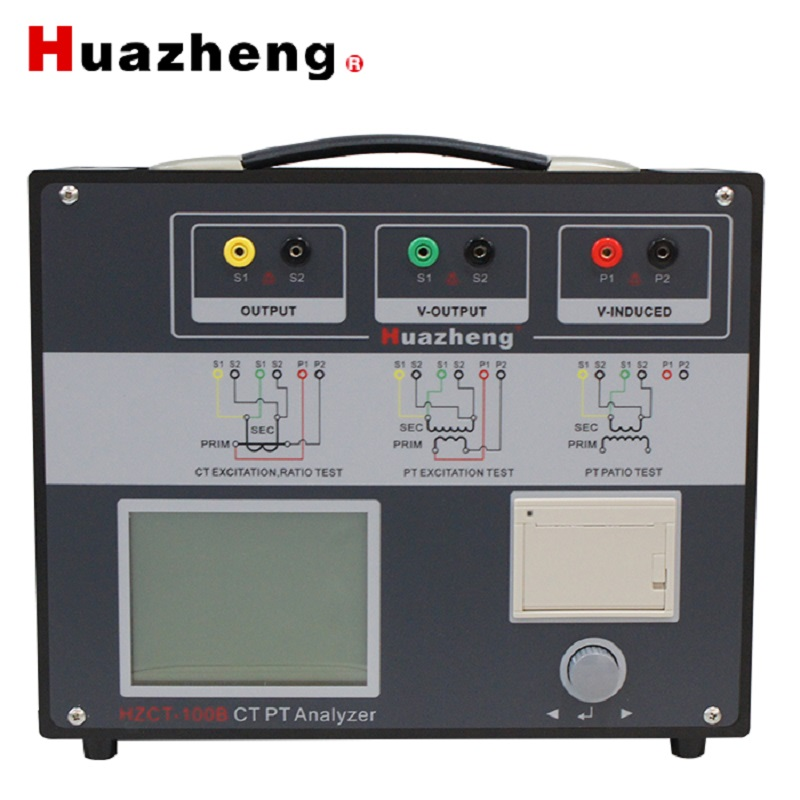HZCT-100B CT PT Analyzer CT PT Excitation Characteristic Analyzer Automatic CT PT Measuring Analyser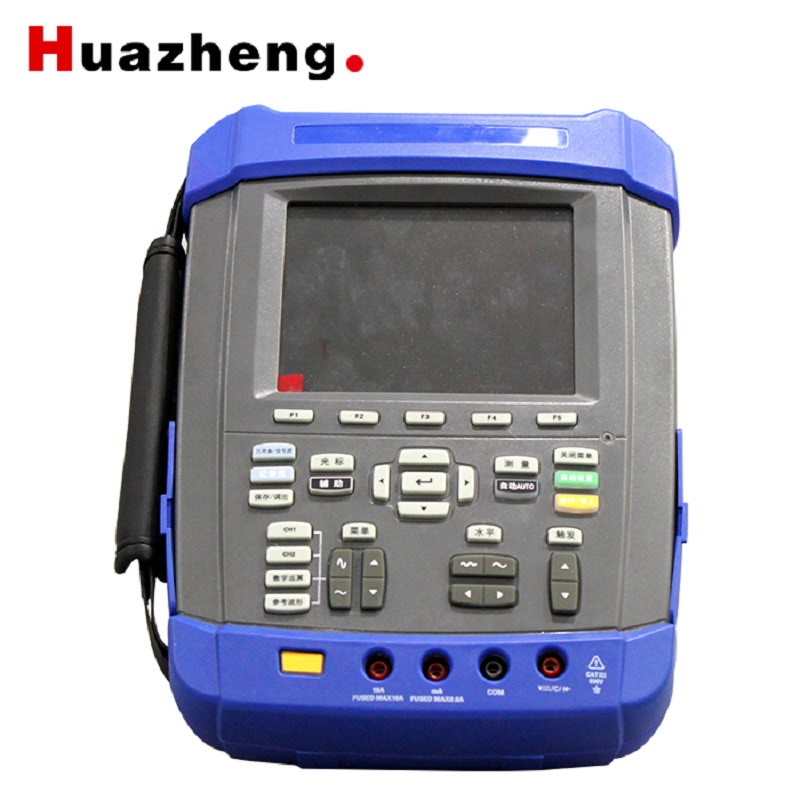HZ-9003C partial discharge tester partial discharge free detection testing transformer partial discharging meter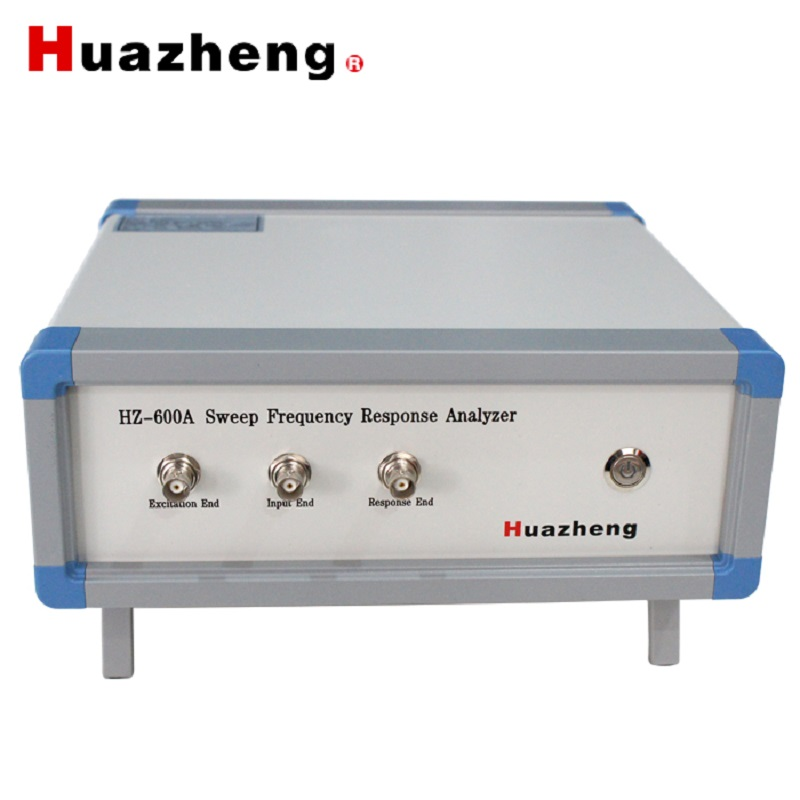HZ-600A Sweep Frequency Response Analyzer Winding Deformation Test Set Transformer SFRA Sweep Frequency Response Analyzer